# NumPy Array manipulation: unique() function

## numpy.unique() function

The unique() function is used to find the unique elements of an array.Returns the sorted unique elements of an array. There are three optional outputs in addition to the unique elements:

• the indices of the input array that give the unique values
• the indices of the unique array that reconstruct the input array
• the number of times each unique value comes up in the input array

Syntax:

`numpy.unique(filt, trim='fb')`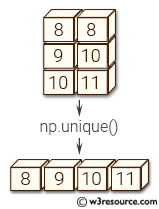Version: 1.15.0

Parameter:

Name Description Required /
Optional
ar Input array. Unless axis is specified, this will be flattened if it is not already 1-D. Required
return_index If True, also return the indices of ar (along the specified axis, if provided, or in the flattened array) that result in the unique array. Optional
return_inverse If True, also return the indices of the unique array (for the specified axis, if provided) that can be used to reconstruct ar. Optional
return_counts If True, also return the number of times each unique item appears in ar. Optional
axis The axis to operate on. If None, ar will be flattened. If an integer, the subarrays indexed by the given axis will be flattened and treated as the elements of a 1-D array with the dimension of the given axis, see the notes for more details. Object arrays or structured arrays that contain objects are not supported if the axis kwarg is used. The default is None. Optional

Return value:

unique : ndarray - The sorted unique values.
unique_indices : ndarray, optional - The indices of the first occurrences of the unique values in the original array. Only provided if return_index is True.
unique_inverse : ndarray, optional - The indices to reconstruct the original array from the unique array. Only provided if return_inverse is True.
unique_counts : ndarray, optional - The number of times each of the unique values comes up in the original array. Only provided if return_counts is True.
New in version 1.9.0.

Example-1: numpy.unique() function

``````>>> import numpy as np
>>> np.unique([0,1,2,0,2,3,4,3,0,4])
array([0, 1, 2, 3, 4])
``````

Pictorial Presentation: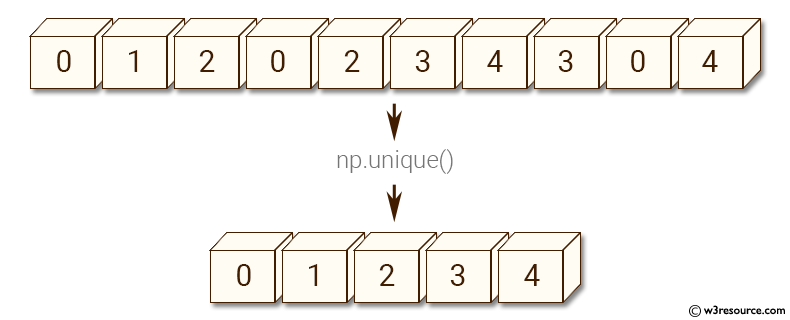Example-2: numpy.unique() function

``````>>> import numpy as np
>>> x = np.array([[1, 1], [2,3], [3,4]])
>>> np.unique(x)
array([1, 2, 3, 4])
``````

Pictorial Presentation: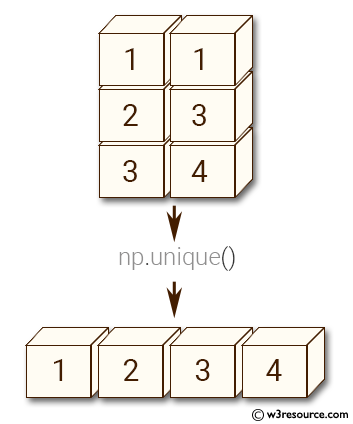Example-3: numpy.unique() function

``````>>> import numpy as np
>>> x = np.array(['o', 'p', 'y', 't', 'h', 'o', 'p'])
>>> u, indices = np.unique(x, return_index=True)
>>> u
array(['h', 'o', 'p', 't', 'y'],
dtype='<U1')
>>> indices
array([4, 0, 1, 3, 2])
>>> x[indices]
array(['h', 'o', 'p', 't', 'y'],
dtype='<U1')
``````

Example-4: numpy.unique() function

``````>>> import numpy as np
>>> x = np.array([0, 1, 2, 5, 2, 6, 5, 2, 3, 1])
>>> u, indices = np.unique(x, return_inverse=True)
>>> u
array([0, 1, 2, 3, 5, 6])
``````

Pictorial Presentation: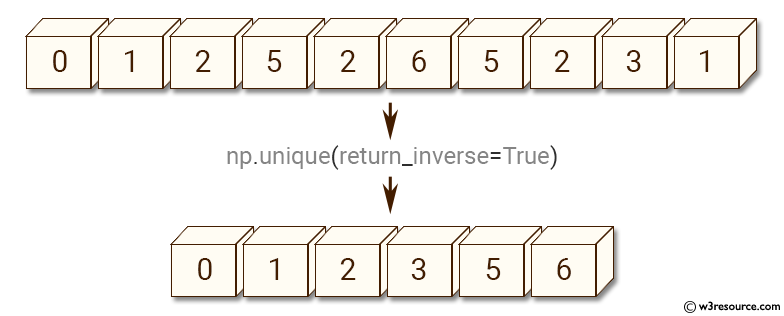Example-5: numpy.unique() function

``````>>> import numpy as np
>>> x = np.array([0, 1, 2, 5, 2, 6, 5, 2, 3, 1])
>>> indices
array([0, 1, 2, 4, 2, 5, 4, 2, 3, 1])
>>> u[indices]
array([0, 1, 2, 5, 2, 6, 5, 2, 3, 1])
``````

Example-6: numpy.unique() function

``````>>> import numpy as np
>>> x = np.array([1,2,5,3,4,2,3,2,5,4])
>>> u, indices = np.unique(x, return_inverse=True)
>>> u
array([1, 2, 3, 4, 5])
``````

Pictorial Presentation: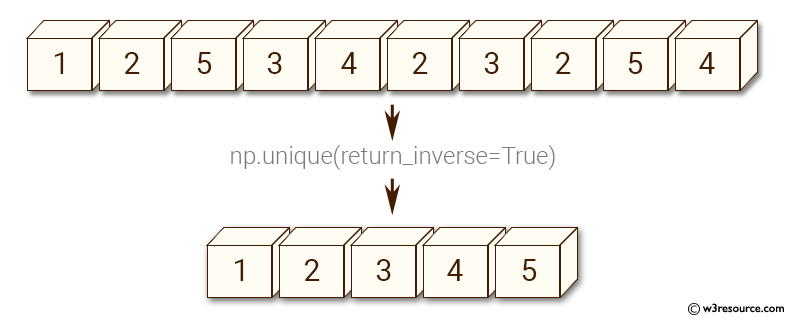Example-7: numpy.unique() function

``````>>> import numpy as np
>>> x = np.array([1,2,5,3,4,2,3,2,5,4])
>>> u, indices = np.unique(x, return_inverse=True)
>>> indices
array([0, 1, 4, 2, 3, 1, 2, 1, 4, 3])
>>> u[indices]
array([1, 2, 5, 3, 4, 2, 3, 2, 5, 4])
``````

Python - NumPy Code Editor:

Previous: trim_zeros()
Next: Rearrangeing elements flip()

﻿